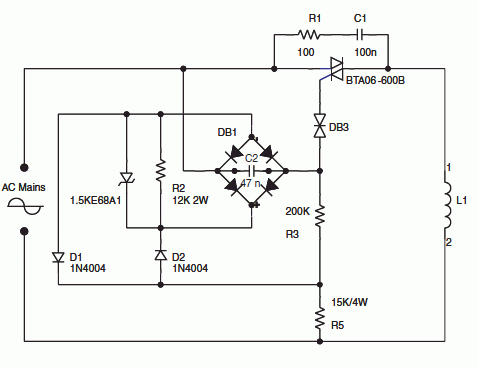# 220V to 110 V step-down circuit

The schematic shows a 220V to 110V step-down circuit used to approximately divide by two the rms voltage between the line input and the inductive load (L1). C2 and R3 provide a turn-on delay of approximately 7 ms.
The diode bridge, with diodes D1 and D2 and R2 resistor are used to discharge C2 at each line zero voltage. This resets the voltage charge at each new half line cycle, and keeps the same turn-on delay.
The Transil reduces the power dissipation through R3.

Synchronization by the mains voltage.  220/110 V step-down circuit